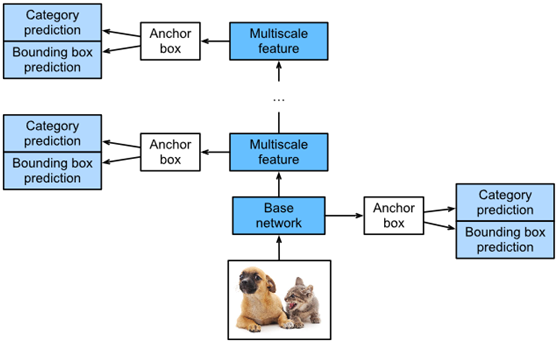# Single Shot Multibox Detection (SSD)实战（上）

Single Shot Multibox Detection (SSD)实战（上）

1. ModelFig. 1 The SSD is composed of a base network block and several multiscale feature blocks connected in a series.

1.1. Category Prediction Layer

%matplotlib inline

from d2l
import mxnet as d2l

from mxnet
import autograd, gluon, image, init, np, npx

from mxnet.gluon
import nn

npx.set_np()

def cls_predictor(num_anchors, num_classes):

return
nn.Conv2D(num_anchors * (num_classes + 1), kernel_size=3,

1.2. Bounding Box Prediction Layer

def bbox_predictor(num_anchors):

return nn.Conv2D(num_anchors * 4, kernel_size=3, padding=1)

1.3. Concatenating Predictions for Multiple Scales

def forward(x, block):

block.initialize()

return


block(x)

Y1 = forward(np.zeros((2, 8, 20, 20)), cls_predictor(5,10))

Y2 = forward(np.zeros((2, 16, 10, 10)), cls_predictor(3,10))

(Y1.shape, Y2.shape)

((2, 55, 20, 20), (2, 33, 10, 10))

def flatten_pred(pred):

return


npx.batch_flatten(pred.transpose(0, 2, 3, 1))

def concat_preds(preds):

return np.concatenate([flatten_pred§ for
p in preds], axis=1)

concat_preds([Y1, Y2]).shape

(2, 25300)

1.4. Height and Width Downsample Block

def down_sample_blk(num_channels):

blk = nn.Sequential()

for _ in range(2):

nn.BatchNorm(in_channels=num_channels),

nn.Activation('relu'))



return blk

forward(np.zeros((2, 3, 20, 20)), down_sample_blk(10)).shape

(2, 10, 10, 10)

1.5. Base Network Block

def base_net():

blk = nn.Sequential()

for num_filters in [16, 32, 64]:

return blk


forward(np.zeros((2, 3, 256, 256)), base_net()).shape

(2, 64, 32, 32)

1.6. The Complete Model

SSD型号共包含五个模块。每个模块输出一个特征映射，用于生成锚框并预测这些锚框的类别和偏移量。第一个模块是基本网络块，模块2到4个是高度和宽度下采样块，第五个模块是一个全局最大池化层，它将高度和宽度减小到1。因此，模块2到5都是图1所示的多尺度特征块。

def get_blk(i):

if i == 0:

blk = base_net()

elif i == 4:

blk = nn.GlobalMaxPool2D()

else:

blk = down_sample_blk(128)


return blk

def blk_forward(X, blk, size, ratio, cls_predictor, bbox_predictor):

Y = blk(X)

anchors = npx.multibox_prior(Y, sizes=size, ratios=ratio)

cls_preds = cls_predictor(Y)

bbox_preds = bbox_predictor(Y)

return (Y, anchors, cls_preds, bbox_preds)


sizes = [[0.2, 0.272], [0.37, 0.447], [0.54, 0.619], [0.71, 0.79],

     [0.88, 0.961]]


ratios = [[1, 2, 0.5]] * 5

num_anchors = len(sizes) + len(ratios) – 1

class TinySSD(nn.Block):

def __init__(self, num_classes, **kwargs):

super(TinySSD, self).__init__(**kwargs)

self.num_classes = num_classes

for i in range(5):

# The assignment statement is self.blk_i = get_blk(i)

setattr(self, 'blk_%d' % i, get_blk(i))

setattr(self, 'cls_%d' % i, cls_predictor(num_anchors,

num_classes))

setattr(self, 'bbox_%d' % i, bbox_predictor(num_anchors))


def forward(self, X):

    anchors, cls_preds, bbox_preds = [None] * 5, [None] * 5, [None] * 5

for i in range(5):

# getattr(self, 'blk_%d' % i) accesses self.blk_i

X, anchors[i], cls_preds[i], bbox_preds[i] = blk_forward(

X, getattr(self, 'blk_%d' % i), sizes[i], ratios[i],

getattr(self, 'cls_%d' % i), getattr(self, 'bbox_%d' % i))

# In the reshape function, 0 indicates that the batch size remains

# unchanged

anchors = np.concatenate(anchors, axis=1)

cls_preds = concat_preds(cls_preds)

cls_preds = cls_preds.reshape(

cls_preds.shape, -1, self.num_classes + 1)

bbox_preds = concat_preds(bbox_preds)

return anchors, cls_preds, bbox_preds


net = TinySSD(num_classes=1)

net.initialize()

X = np.zeros((32, 3, 256, 256))

anchors, cls_preds, bbox_preds = net(X)

print(‘output anchors:’, anchors.shape)

print(‘output class preds:’, cls_preds.shape)

print(‘output bbox preds:’, bbox_preds.shape)

output anchors: (1, 5444, 4)

output class preds: (32, 5444, 2)

output bbox preds: (32, 21776)

06-06255606-302904
07-131万+
11-011万+
04-241140
06-25858
12-19368
09-063847
09-199万+
09-245043
04-183741
12-312769
11-294164
10-153552
11-077173
01-141万+
09-082027